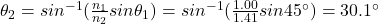## Set the top medium to AIR. Set the second medium to CUSTOM. Move the slider for the index of refraction to 1.41. 2) Use Snell’s La

Question

Set the top medium to AIR. Set the second medium to CUSTOM. Move the slider for the index of refraction to 1.41.
2) Use Snell’s Law to find the following (show your work): If θi = 45°, what is the value of θr?

in progress 0
1 month 2021-08-01T07:34:57+00:00 1 Answers 3 views 0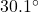Explanation:

Refraction is a phenomenon that occurs when light passes through the interface between two mediums.

When this occurs, the ray of light changes speed and also changes direction, according to Snell’s Law: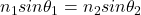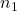is the index of refraction of the 1st medium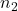is the index of refraction of the 2nd medium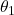is the angle of incidence (the angle between the incident ray and the normal to the boundary)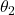is the angle of refraction (the angle between the refracted ray and the normal to the boundary)

For this problem we have: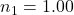is the index of refraction of the 1st medium (air)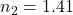is the  index of refraction of the 2nd medium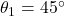is the angle of incidence

Solving for the angle of refraction, we find: